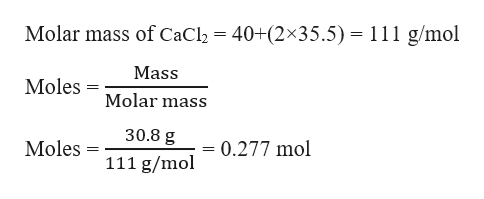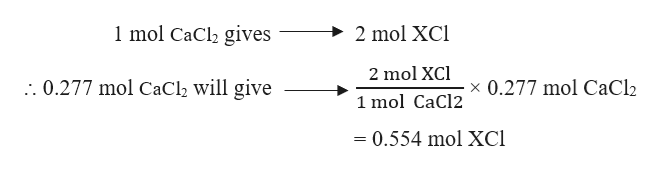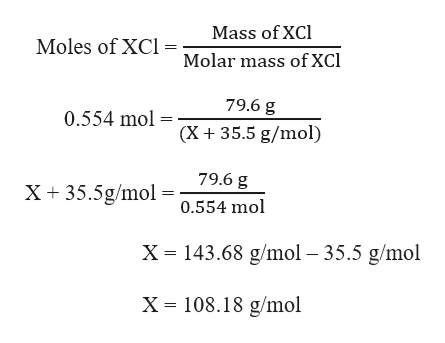Consider the following unbalanced reaction:XNO3 (aq) + CaCl2 (aq) -> XCl (s) + Ca(NO3)2 (aq)If 30.8 g of CaCl2 produced 79.6 g of XCl, determine the identity of X. Quantify your response.

Question

Consider the following unbalanced reaction:

XNO3 (aq) + CaCl(aq) -> XCl (s) + Ca(NO3)(aq)

If 30.8 g of CaCl2 produced 79.6 g of XCl, determine the identity of X. Quantify your response.

Step 1

Given,
Mass of CaCl2 = 30.8 g
Mass of XCl = 79.6 g

Moles of CaCl2 can be calculated as:help_outlineImage TranscriptioncloseMolar mass of CaCl2 40+(2x35.5) 111 g/mol Mass Moles Molar mass 30.8 g Moles 0.277 mol _ 111 g/mol fullscreen
Step 2

The balanced reaction can be written as:

2 XNO3 (aq) + CaCl(aq) -------> 2 XCl (s) + Ca(NO3)(aq)

From the reaction, it is evident that:help_outlineImage Transcriptionclose1 mol CaCl2 gives 2 mol XCI 2 mol XCl . 0.277 mol CaCl2 will give x 0.277 mol CaCl2 1 mol CaCl2 = 0.554 mol XCl fullscreen
Step 3

Moles of XCl can be...help_outlineImage TranscriptioncloseMass of XCl Moles of XC Molar mass of XCl 79.6 g 0.554 mol (X 35.5 g/mol) 79.6 g X35.5g/mol0.554 mol X 143.68 g/mol 35.5 g/mol X =108.18 g/mol fullscreen

Want to see the full answer?

See Solution

Want to see this answer and more?

Our solutions are written by experts, many with advanced degrees, and available 24/7

See Solution
Tagged in

General Chemistry